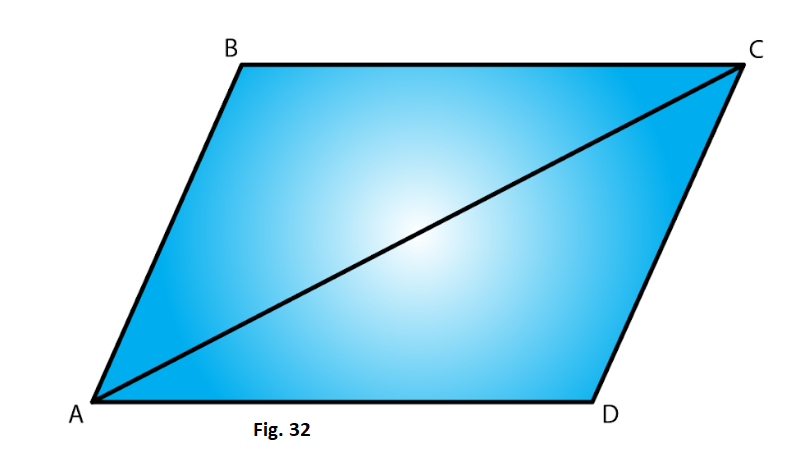# RD Sharma Solutions For Class 7 Maths Chapter 16 Congruence Exercise 16.3

The study materials of RD Sharma Solutions for Class 7 Maths Exercise 16.3 of Chapter 16, in the form of PDF, is available here. Students who want to score high in Maths can download these materials from the given links. Our expert team have formulated RD Sharma Solutions for Class 7 to help and fulfill the dreams of students. This exercise has thirty questions based on SAS congruence condition along with proof.

## Download the PDF of RD Sharma Solutions For Class 7 Maths Chapter 16 – Congruence Exercise 16.3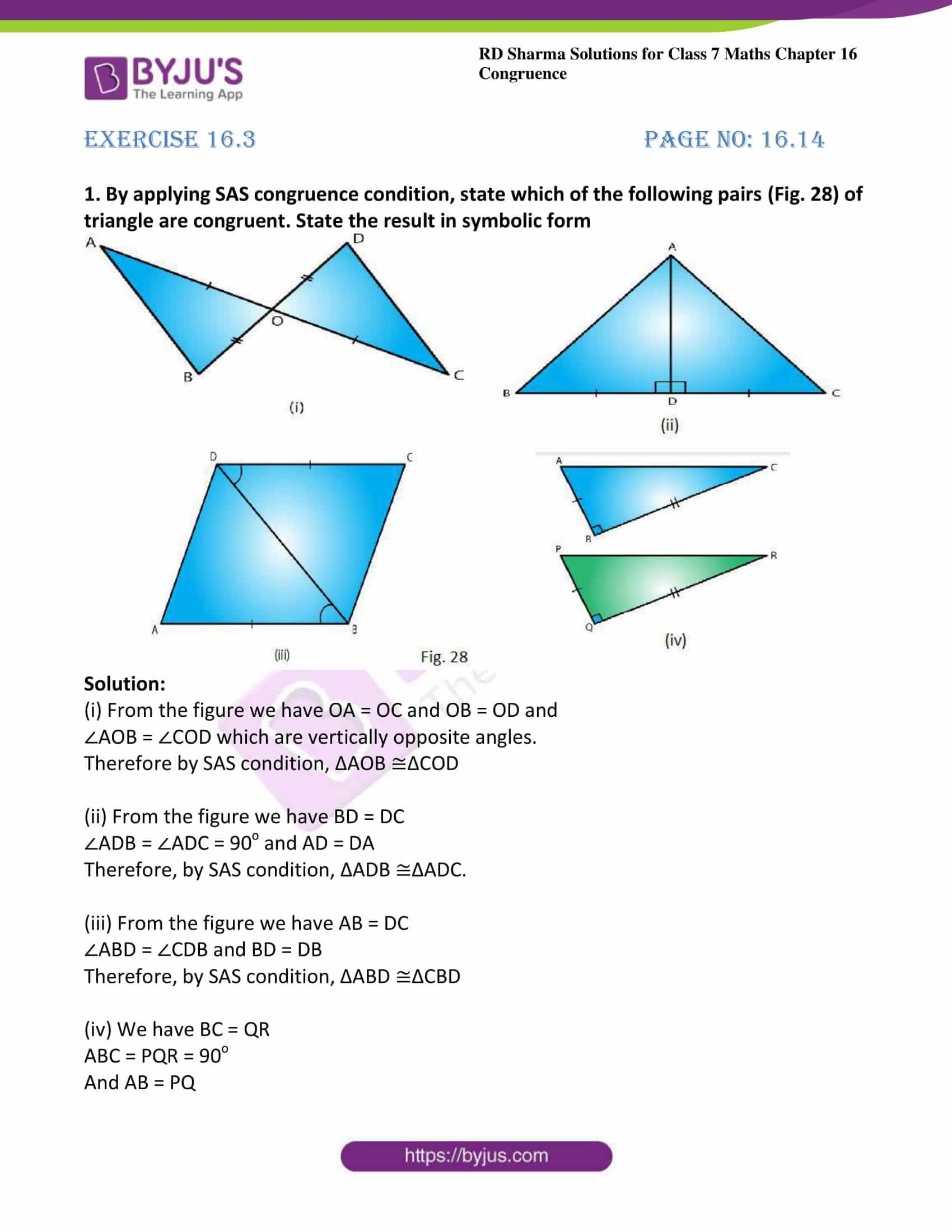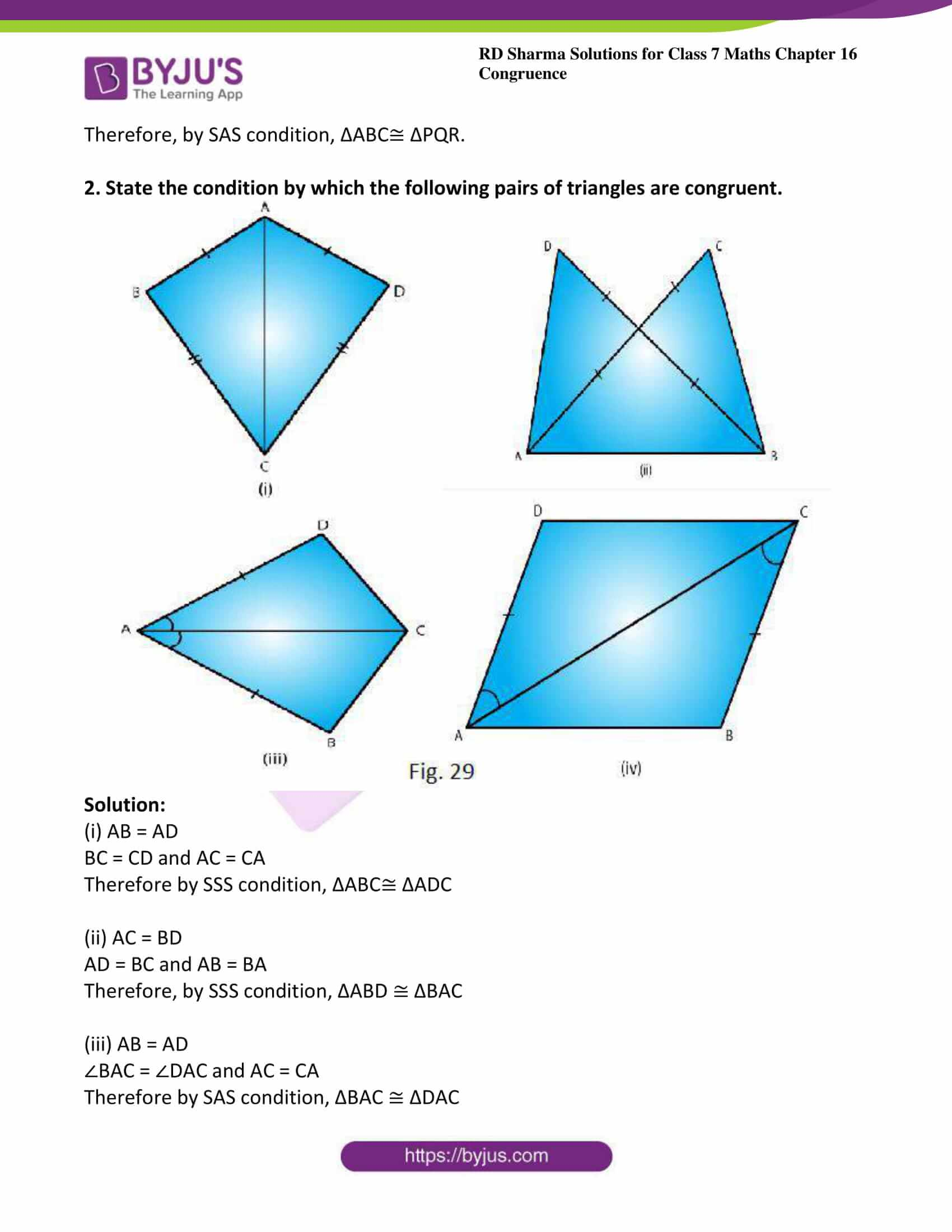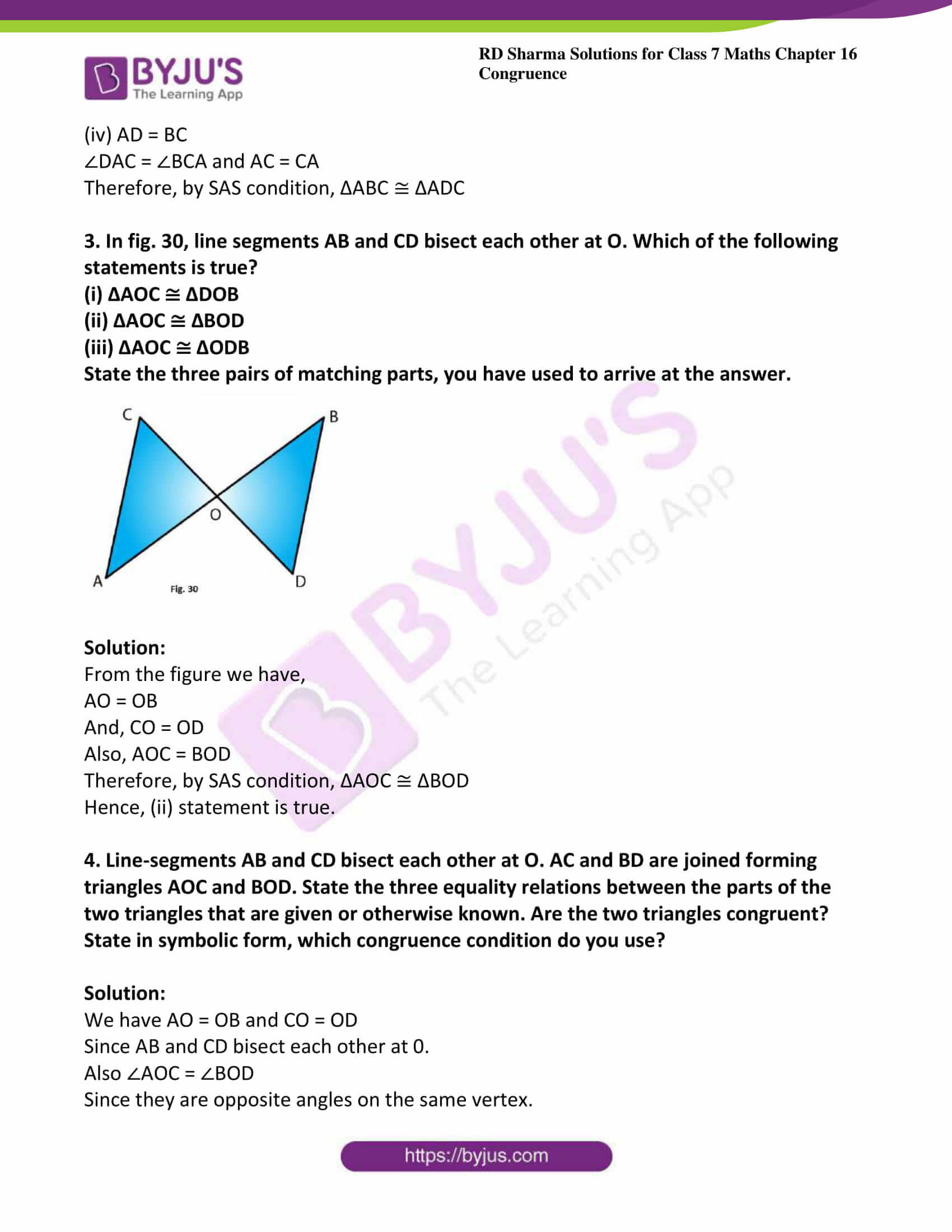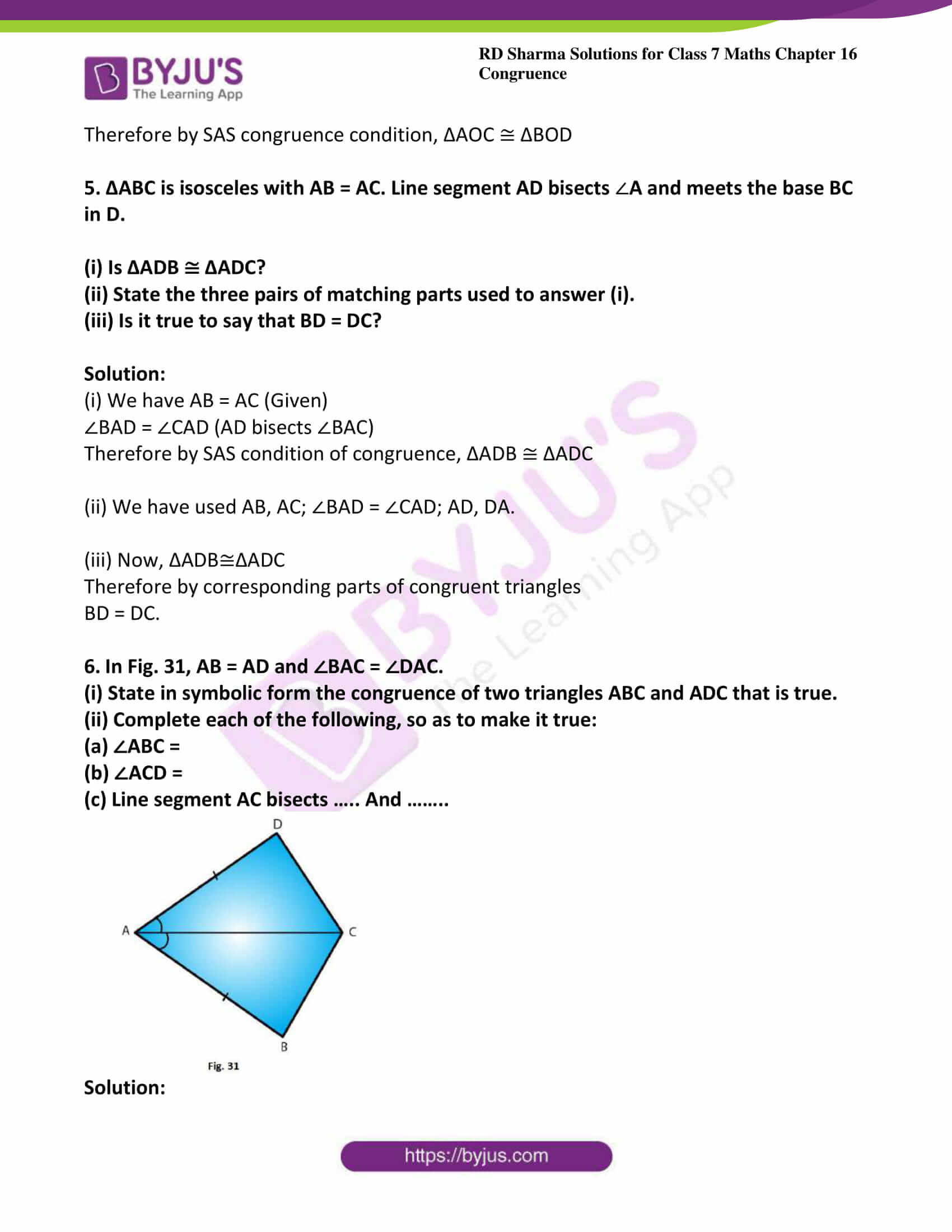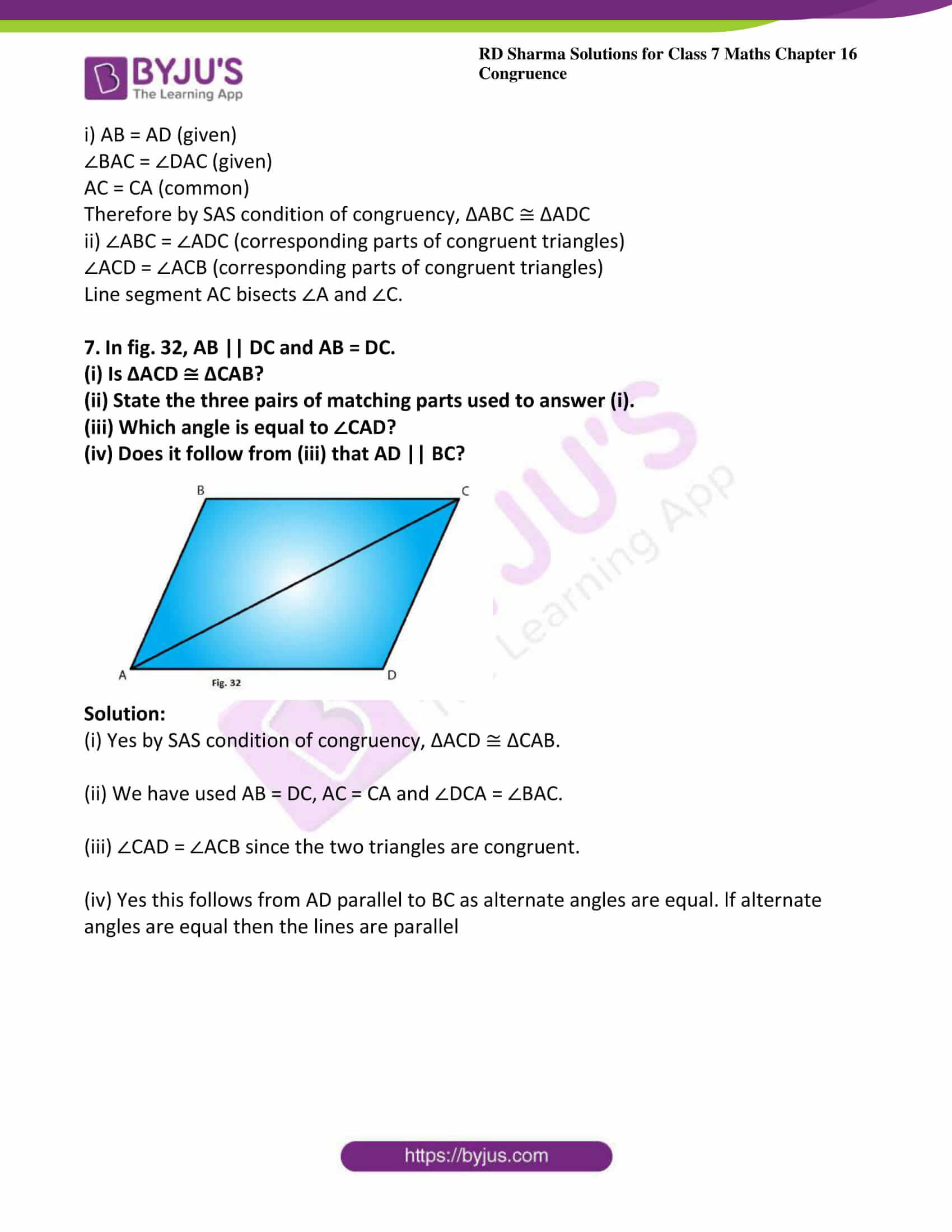### Access answers to Maths RD Sharma Solutions For Class 7 Chapter 16 – Congruence Exercise 16.3

Exercise 16.3 Page No: 16.14

1. By applying SAS congruence condition, state which of the following pairs (Fig. 28) of triangle are congruent. State the result in symbolic form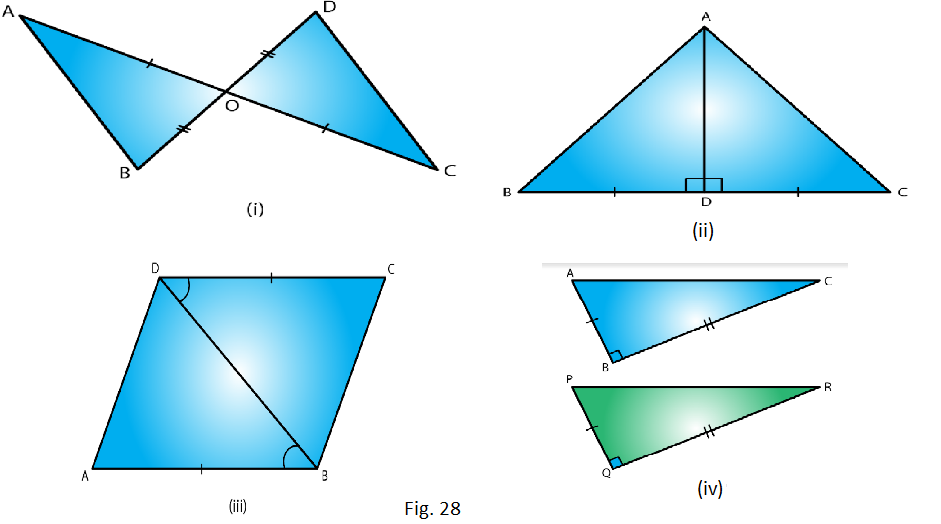Solution:

(i) From the figure we have OA = OC and OB = OD and

∠AOB = ∠COD which are vertically opposite angles.

Therefore by SAS condition, ΔAOB ≅ΔCOD

(ii) From the figure we have BD = DC

(iii) From the figure we have AB = DC

∠ABD = ∠CDB and BD = DB

Therefore, by SAS condition, ΔABD ≅ΔCBD

(iv) We have BC = QR

ABC = PQR = 90o

And AB = PQ

Therefore, by SAS condition, ΔABC≅ ΔPQR.

2. State the condition by which the following pairs of triangles are congruent.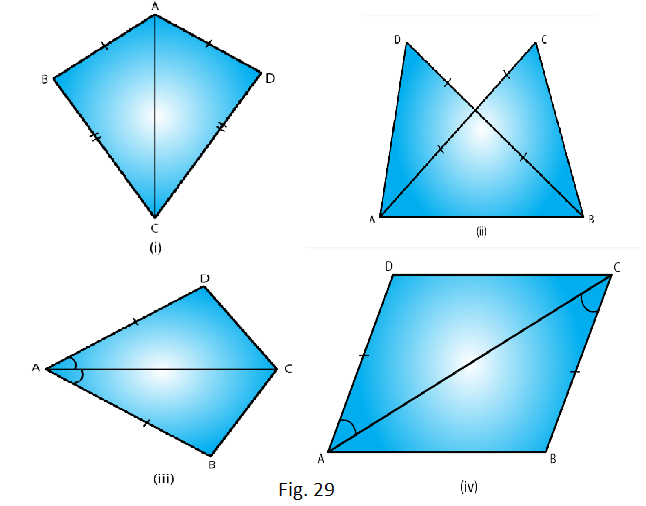Solution:

BC = CD and AC = CA

Therefore by SSS condition, ΔABC≅ ΔADC

(ii) AC = BD

AD = BC and AB = BA

Therefore, by SSS condition, ΔABD ≅ ΔBAC

∠BAC = ∠DAC and AC = CA

Therefore by SAS condition, ΔBAC ≅ ΔDAC

∠DAC = ∠BCA and AC = CA

Therefore, by SAS condition, ΔABC ≅ ΔADC

3. In fig. 30, line segments AB and CD bisect each other at O. Which of the following statements is true?

(i) ΔAOC ≅ ΔDOB

(ii) ΔAOC ≅ ΔBOD

(iii) ΔAOC ≅ ΔODB

State the three pairs of matching parts, you have used to arrive at the answer.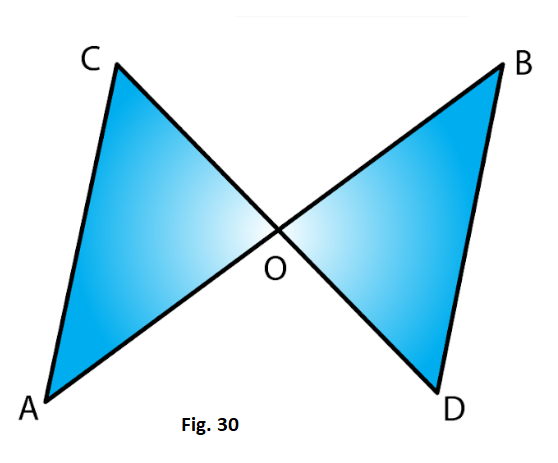Solution:

From the figure we have,

AO = OB

And, CO = OD

Also, AOC = BOD

Therefore, by SAS condition, ΔAOC ≅ ΔBOD

Hence, (ii) statement is true.

4. Line-segments AB and CD bisect each other at O. AC and BD are joined forming triangles AOC and BOD. State the three equality relations between the parts of the two triangles that are given or otherwise known. Are the two triangles congruent? State in symbolic form, which congruence condition do you use?

Solution:

We have AO = OB and CO = OD

Since AB and CD bisect each other at 0.

Also ∠AOC = ∠BOD

Since they are opposite angles on the same vertex.

Therefore by SAS congruence condition, ΔAOC ≅ ΔBOD

5. ΔABC is isosceles with AB = AC. Line segment AD bisects A and meets the base BC in D.

(ii) State the three pairs of matching parts used to answer (i).

(iii) Is it true to say that BD = DC?

Solution:

(i) We have AB = AC (Given)

Therefore by corresponding parts of congruent triangles

BD = DC.

6. In Fig. 31, AB = AD and ∠BAC = ∠DAC.

(i) State in symbolic form the congruence of two triangles ABC and ADC that is true.

(ii) Complete each of the following, so as to make it true:

(a) ∠ABC =

(b) ∠ACD =

(c) Line segment AC bisects ….. And ……..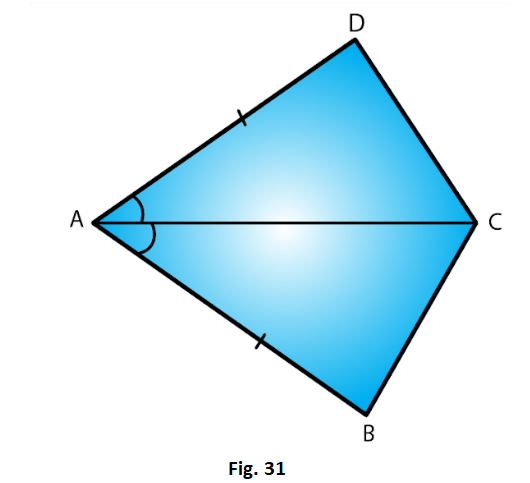Solution:

∠BAC = ∠DAC (given)

AC = CA (common)

Therefore by SAS condition of congruency, ΔABC ≅ ΔADC

ii) ∠ABC = ∠ADC (corresponding parts of congruent triangles)

∠ACD = ∠ACB (corresponding parts of congruent triangles)

Line segment AC bisects ∠A and ∠C.

7. In fig. 32, AB || DC and AB = DC.

(i) Is ΔACD ≅ ΔCAB?

(ii) State the three pairs of matching parts used to answer (i).

(iii) Which angle is equal to ∠CAD?